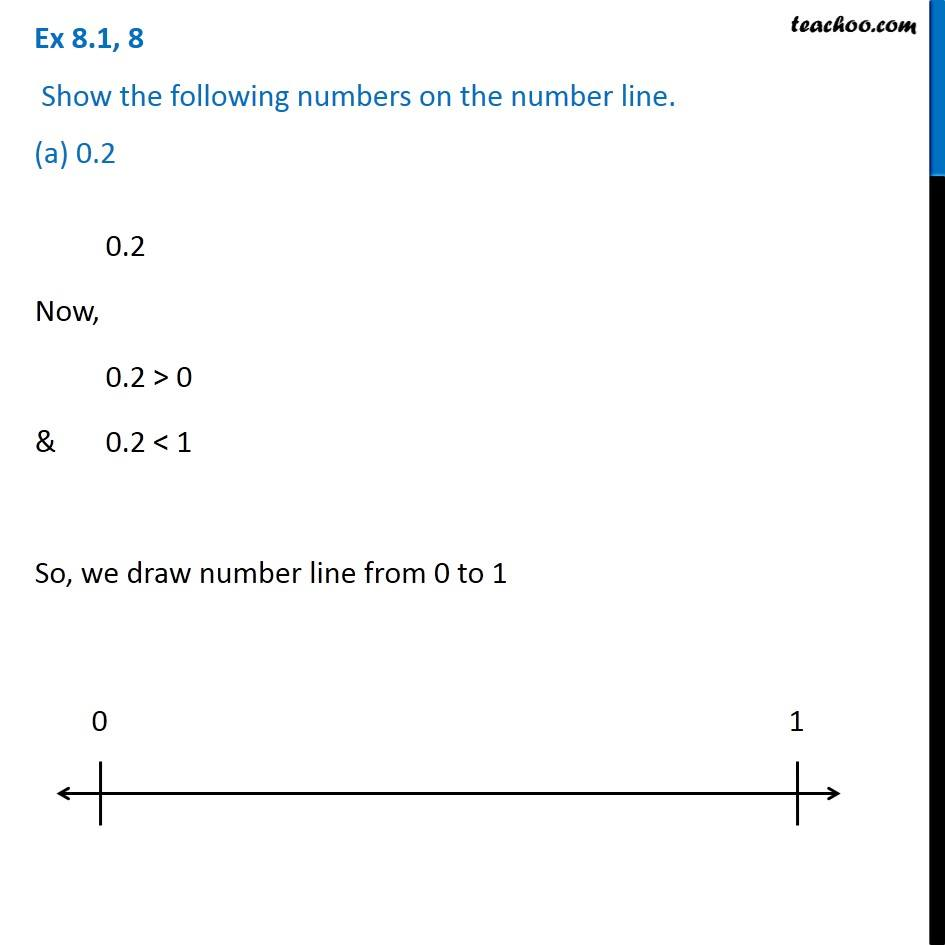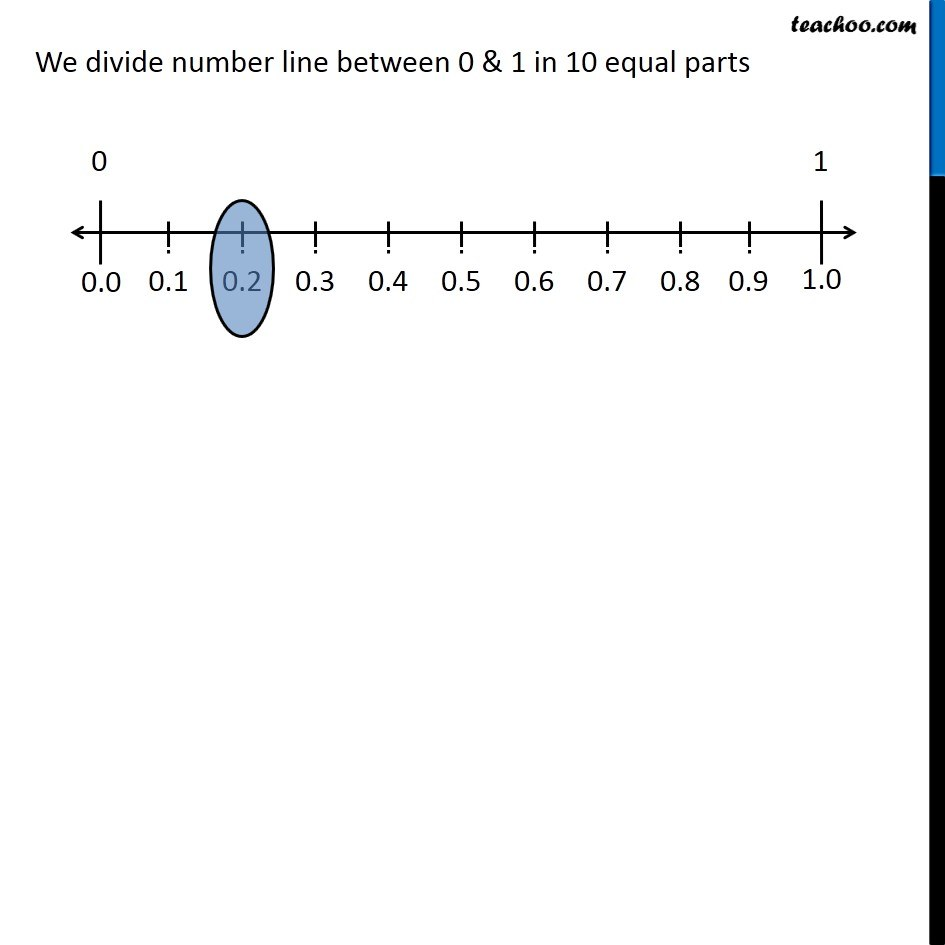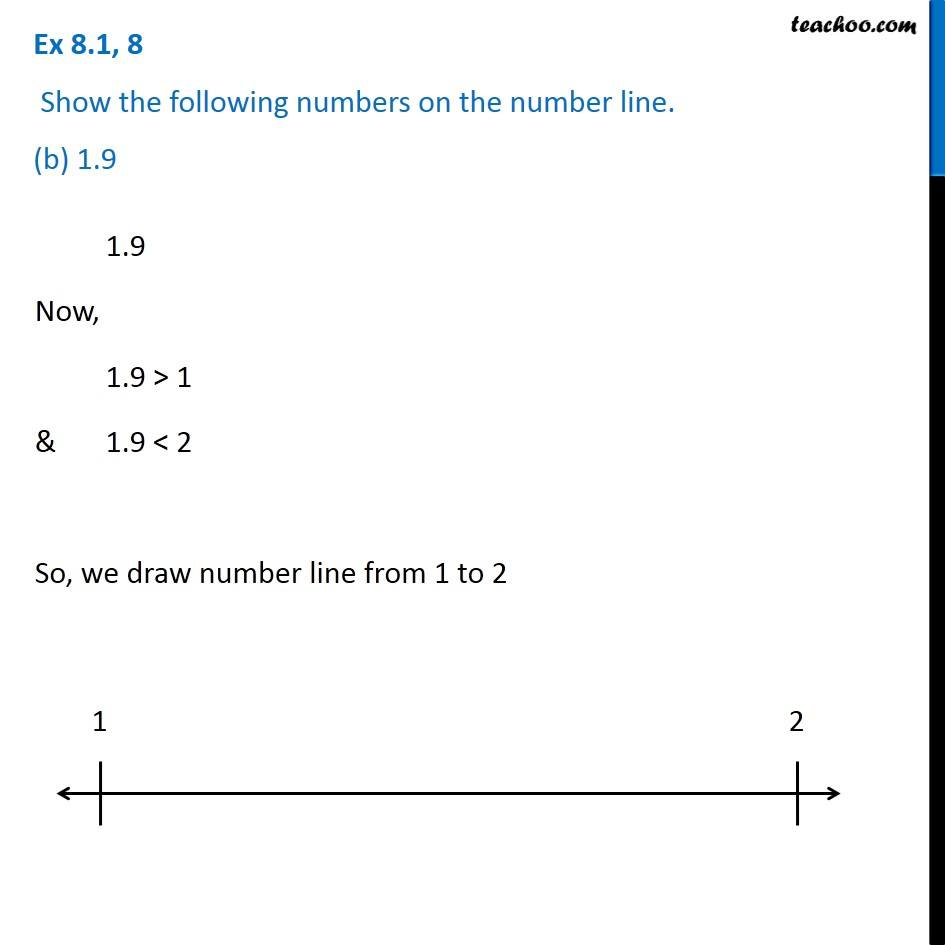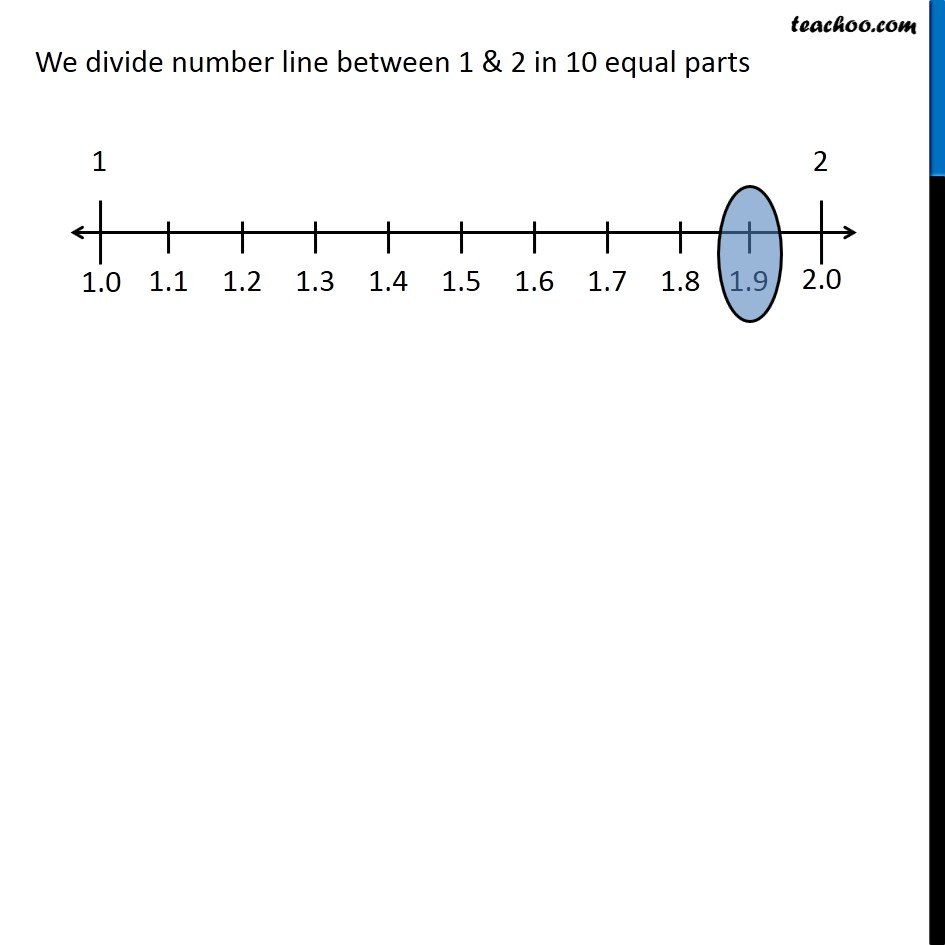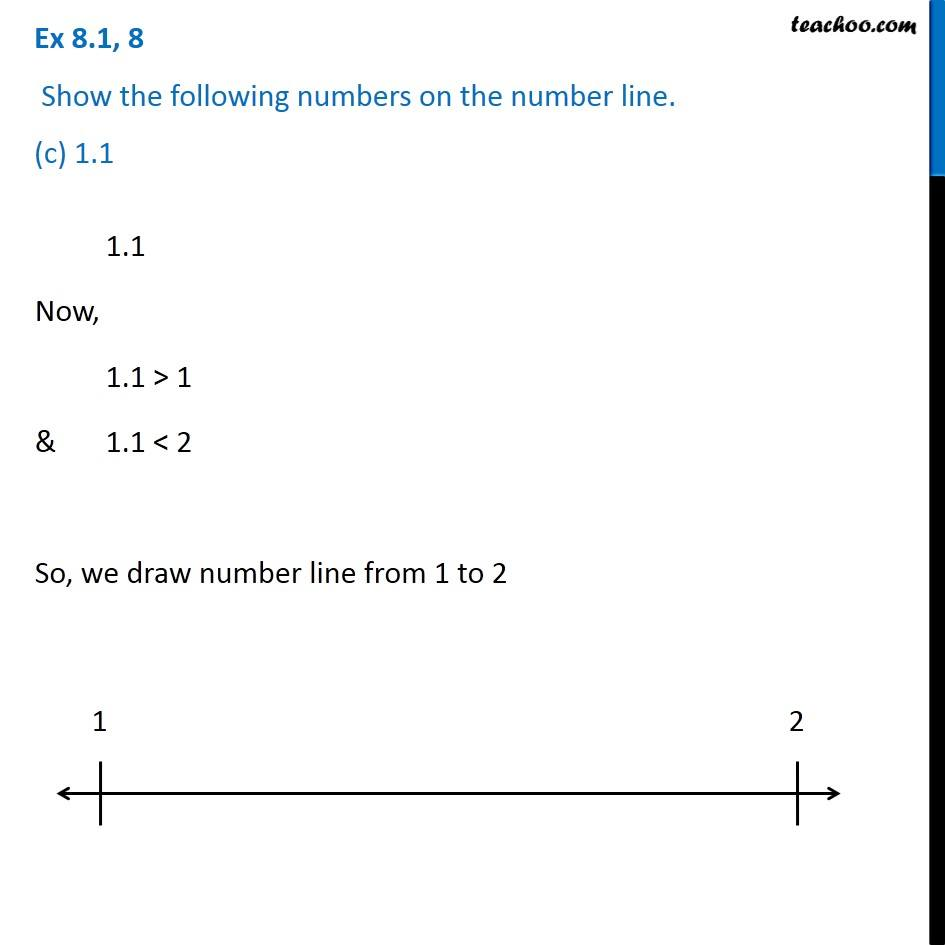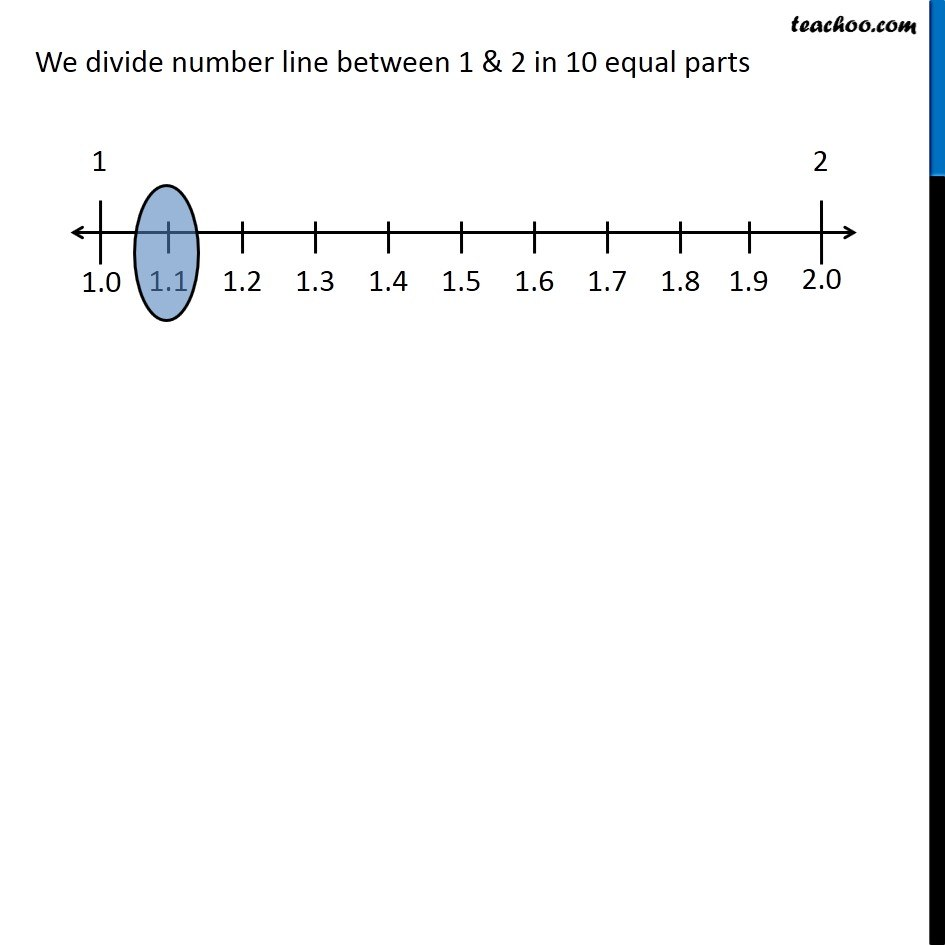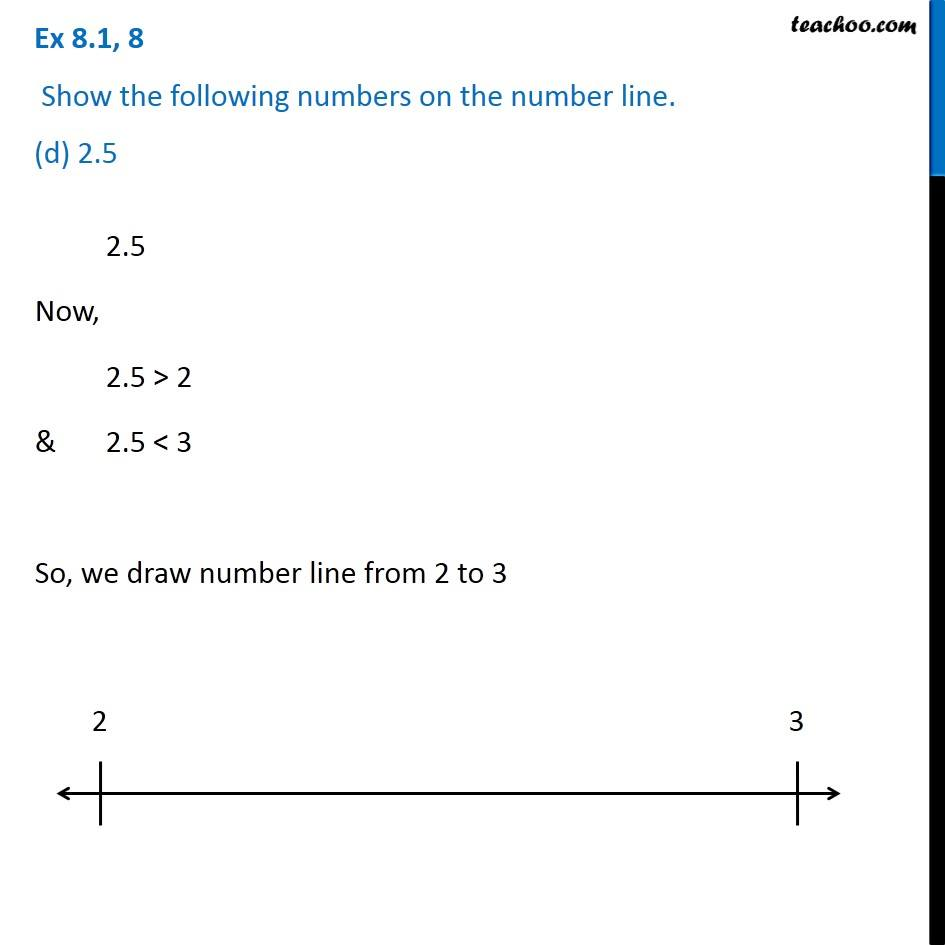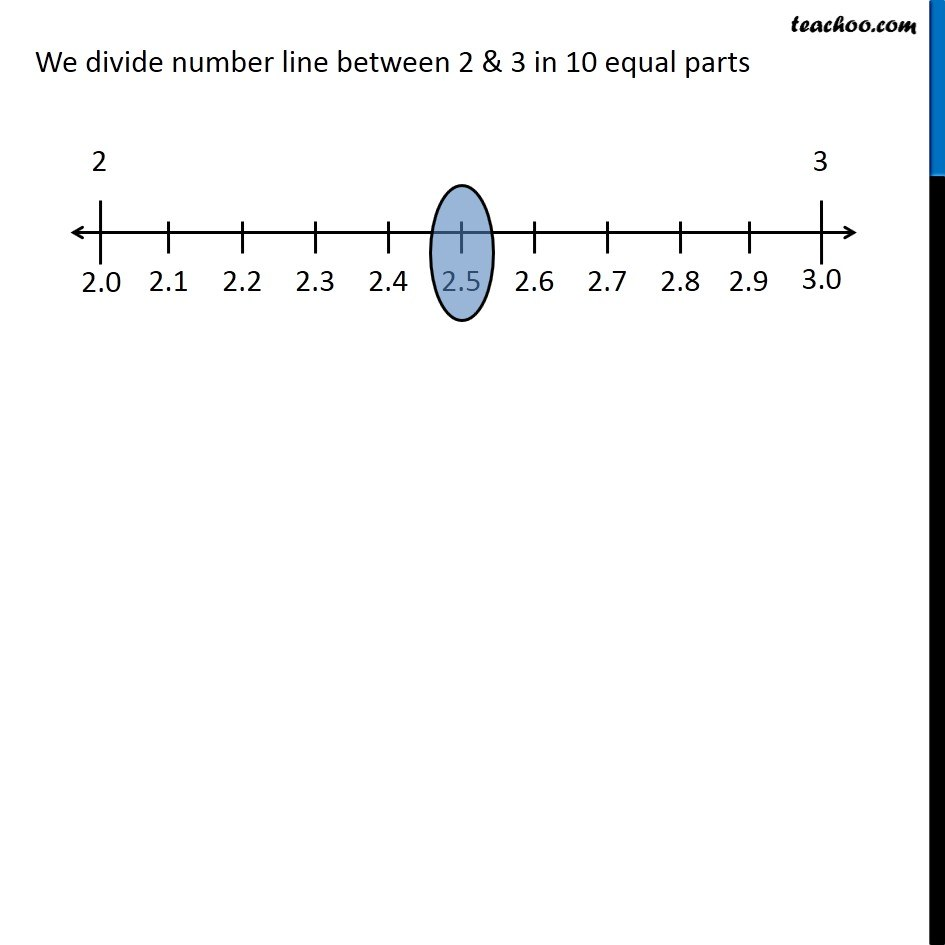Subscribe to our Youtube Channel - https://you.tube/teachoo

1. Chapter 8 Class 6 Decimals
2. Concept wise
3. Decimals in number line

Transcript

Ex 8.1, 8 Show the following numbers on the number line. (a) 0.2 0.2 Now, 0.2 > 0 & 0.2 < 1 So, we draw number line from 0 to 1 We divide number line between 0 & 1 in 10 equal parts Ex 8.1, 8 Show the following numbers on the number line. (b) 1.9 1.9 Now, 1.9 > 1 & 1.9 < 2 So, we draw number line from 1 to 2 1.9 Now, 1.9 > 1 & 1.9 < 2 So, we draw number line from 1 to 2 We divide number line between 1 & 2 in 10 equal parts Ex 8.1, 8 Show the following numbers on the number line. (c) 1.1 1.1 Now, 1.1 > 1 & 1.1 < 2 So, we draw number line from 1 to 2 We divide number line between 1 & 2 in 10 equal parts Ex 8.1, 8 Show the following numbers on the number line. (d) 2.5 2.5 Now, 2.5 > 2 & 2.5 < 3 So, we draw number line from 2 to 3 We divide number line between 2 & 3 in 10 equal parts

Decimals in number line